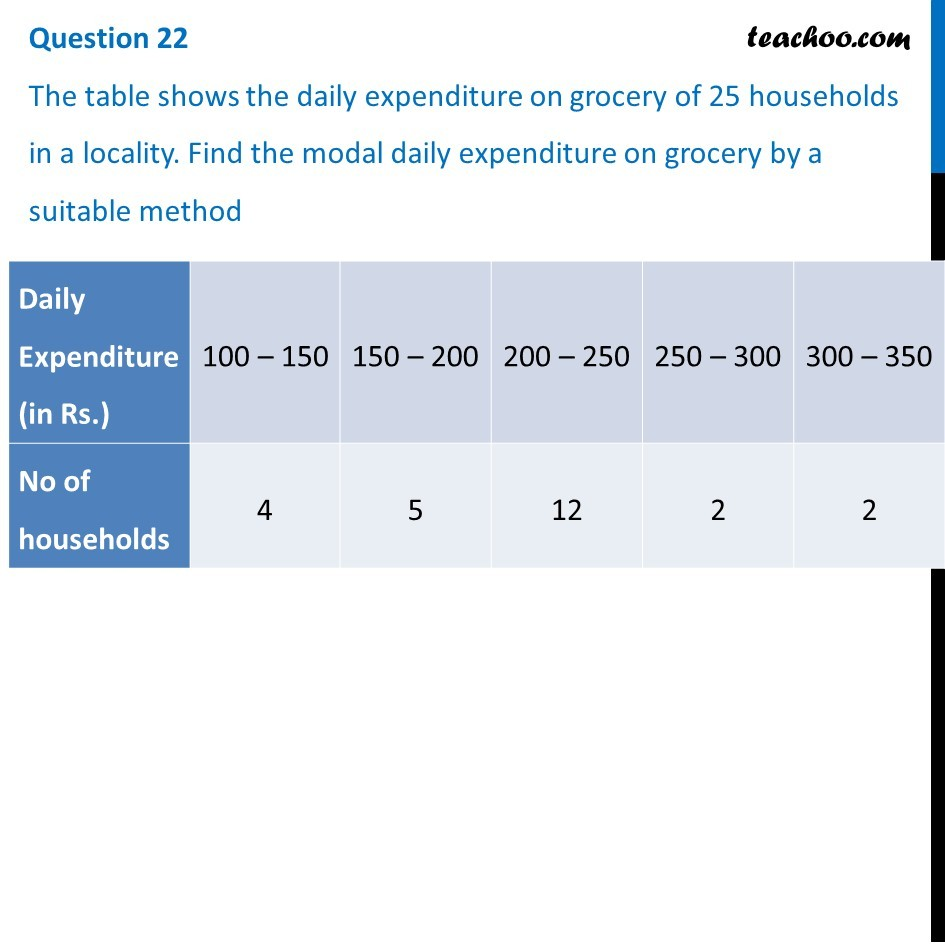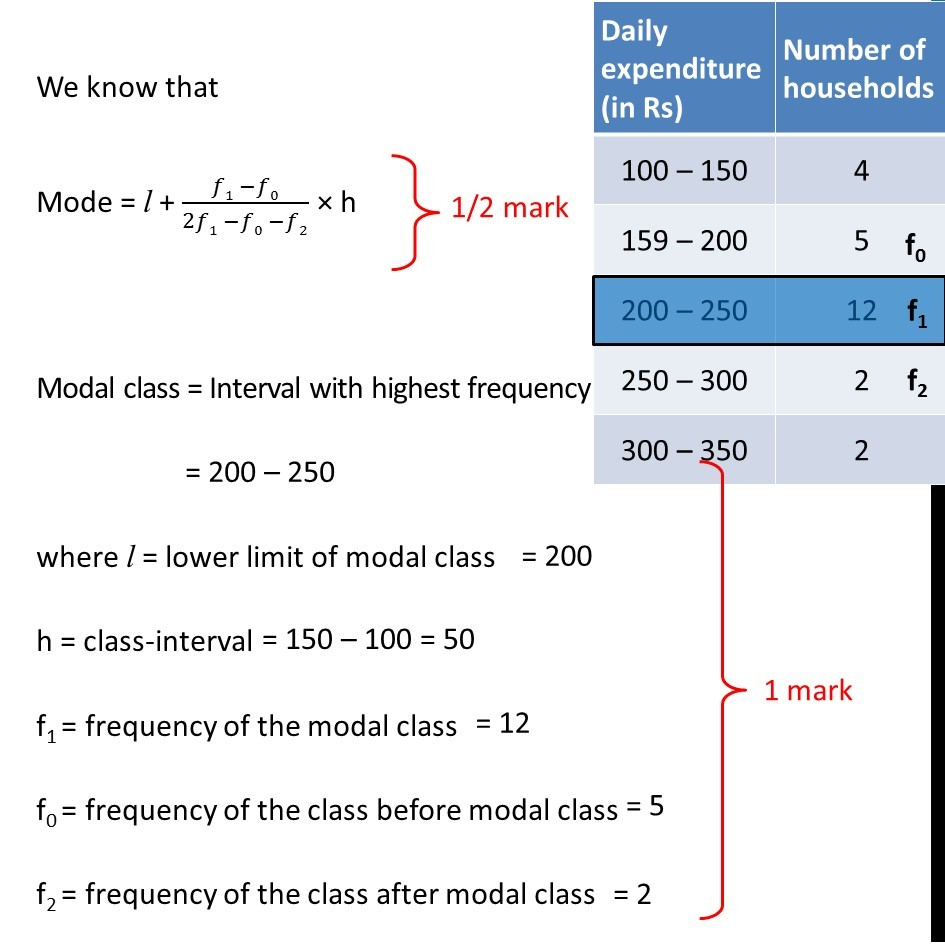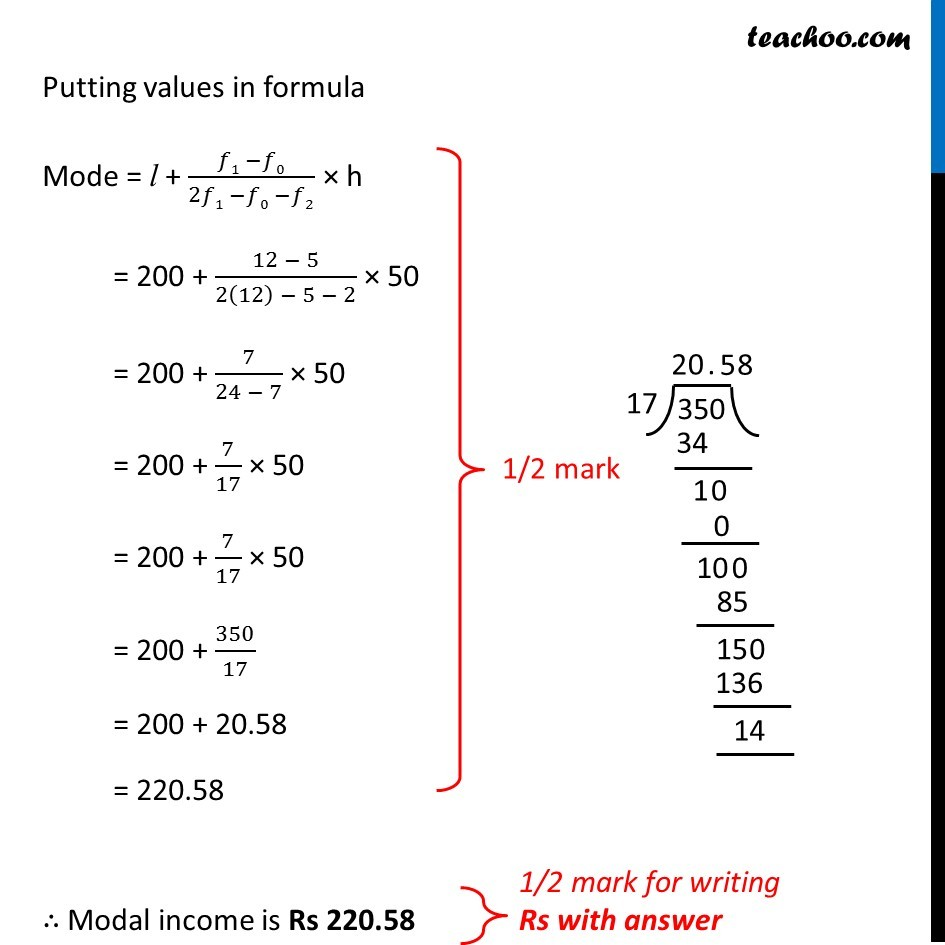CBSE Class 10 Sample Paper for 2019 Boards

Class 10
Solutions of Sample Papers for Class 10 Boards

Question 22

The table shows the daily expenditure on grocery of 25 households in a locality. Find the modal daily expenditure on grocery by a suitable method

 Daily Expenditure (in Rs .) 100 – 150 150 – 200 200 – 250 250 – 300 300 – 350 No of households 4 5 12 2 2Learn in your speed, with individual attention - Teachoo Maths 1-on-1 Class

### Transcript

Question 22 The table shows the daily expenditure on grocery of 25 households in a locality. Find the modal daily expenditure on grocery by a suitable method We know that Mode = l + (𝑓1 −𝑓0)/(2𝑓1 −𝑓0 −𝑓2) × h Modal class = Interval with highest frequency = 200 – 250 where l = lower limit of modal class h = class-interval = 150 – 100 = 50 f1 = frequency of the modal class = 12 f0 = frequency of the class before modal class = 5 f2 = frequency of the class after modal class = 2 Putting values in formula Mode = l + (𝑓1 −𝑓0)/(2𝑓1 −𝑓0 −𝑓2) × h = 200 + (12 − 5)/(2(12) − 5 − 2) × 50 = 200 + 7/(24 − 7) × 50 = 200 + 7/17 × 50 = 200 + 7/17 × 50 = 200 + 350/17 = 200 + 20.58 = 220.58 ∴ Modal income is Rs 220.58